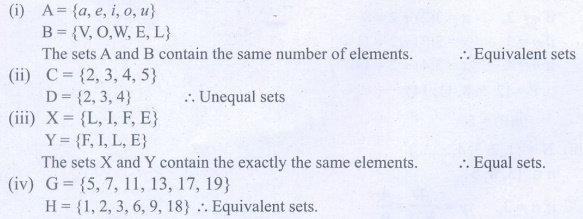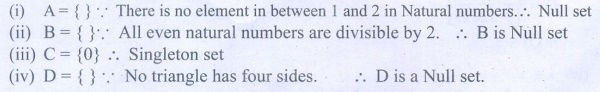Home | | Maths 9th std | Exercise 1.2: Types of Sets

# Exercise 1.2: Types of Sets

Book Back, Exercise, Example Numerical Question with Answers, Solution : Maths : Set Language : Exercise 1.2: Types of Sets

Exercise 1.2

1. Find the cardinal number of the following sets.

(i) M = {p, q, r, s, t, u}

(ii) P = {x : x = 3n+2, nŌłł W and x< 15}

(iii) Q = {y : y = 4/3n, n Ōłł ŌäĢ and 2 < n Ōēż5}

(iv) R = {x : x is an integers, xŌłł Ōäż and ŌĆō5 Ōēż x <5}

(v) S = The set of all leap years between 1882 and 1906.2. Identify the following sets as finite or infinite.

(i) X = The set of all districts in Tamilnadu.

(ii) Y = The set of all straight lines passing through a point.

(iii) A = { x : x Ōłł Ōäż and x <5}

(iv) B = { x : x2ŌĆō5x+6 = 0, x Ōłł ŌäĢ }3. Which of the following sets are equivalent or unequal or equal sets?

(i) A = The set of vowels in the English alphabets.

B = The set of all letters in the word ŌĆ£VOWELŌĆØ

(ii) C= {2,3,4,5}

D = { x : x Ōłł W , 1< x<5}

(iii) X= { x : x is a letter in the word ŌĆ£LIFEŌĆØ}

Y={F,I,L,E}

(iv) G= { x : x is a prime number and 3 < x < 23}

H = { x : x is a divisor of 18}4. Identify the following sets as null set or singleton set.

(i) A = {x : x Ōłł ŌäĢ , 1 < x < 2}

(ii) B = The set of all even natural numbers which are not divisible by 2

(iii) C = {0}.

(iv) D = The set of all triangles having four sides.5. State which pairs of sets are disjoint or overlapping?

(i) A = {f, i, a, s} and B={a, n, f, h, s}

(ii) C = {x : x is a prime number, x >2} and D ={x:x is an even prime number}

(iii) E = {x : x is a factor of 24} and F={x : x is a multiple of 3, x < 30}6. If S = {square, rectangle, circle, rhombus, triangle}, list the elements of the following subset of S.

(i) The set of shapes which have 4 equal sides.

(ii) The set of shapes which have radius.

(iii) The set of shapes in which the sum of all interior angles is 180o.

(iv) The set of shapes which have 5 sides.7. If A = {a, {a, b}}, write all the subsets of A.8. Write down the power set of the following sets:

(i) A = {a, b}

(ii) B = {1, 2, 3}

(iii) D = {p, q, r, s}

(iv) E = Ōłģ9. Find the number of subsets and the number of proper subsets of the following sets.

(i) W = {red, blue, yellow}

(ii) X = { x2 : x Ōłł ŌäĢ , x2 Ōēż 100}.10. If n(A) = 4, find n[P(A)].

(ii) If n(A)=0, find n[P(A)].

(iii) If n[P(A)] = 256, find n(A).Tags : Numerical Problems with Answers, Solution | Set Language | Maths , 9th Maths : UNIT 1 : Set Language
Study Material, Lecturing Notes, Assignment, Reference, Wiki description explanation, brief detail
9th Maths : UNIT 1 : Set Language : Exercise 1.2: Types of Sets | Numerical Problems with Answers, Solution | Set Language | Maths